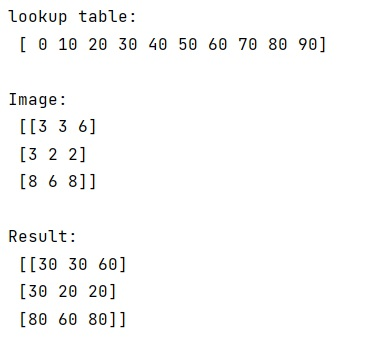# How to apply a lookup table to a large array in NumPy?

Learn about the convenient way to apply a lookup table to a large array in NumPy.
Submitted by Pranit Sharma, on February 26, 2023

NumPy is an abbreviated form of Numerical Python. It is used for different types of scientific operations in python. Numpy is a vast library in python which is used for almost every kind of scientific or mathematical operation. It is itself an array which is a collection of various methods and functions for processing the arrays.

## Applying a lookup table to a large array in NumPy

Suppose we are given an image that we want to read into numpy. We need to apply a lookup table for this image in numpy.

For this purpose, we can just use the image to index the lookup table if the lookup table is 1d.

Let us understand with the help of an example,

## Python code to apply a lookup table to a large array in NumPy

```# Import numpy
import numpy as np

# Creating a lookup table
lookup = np.arange(10) * 10

# Display lookup table
print("lookup table:\n",lookup,"\n")

# Creating an image array
img = np.random.randint(0,9,size=(3,3))

# Display original image
print("Image:\n",img,"\n")

# using img to index lookup
res = lookup[img]

# Display result
print("Result:\n",res)
```

Output:Languages: » C » C++ » C++ STL » Java » Data Structure » C#.Net » Android » Kotlin » SQL
Web Technologies: » PHP » Python » JavaScript » CSS » Ajax » Node.js » Web programming/HTML
Solved programs: » C » C++ » DS » Java » C#
Aptitude que. & ans.: » C » C++ » Java » DBMS
Interview que. & ans.: » C » Embedded C » Java » SEO » HR
CS Subjects: » CS Basics » O.S. » Networks » DBMS » Embedded Systems » Cloud Computing
» Machine learning » CS Organizations » Linux » DOS
More: » Articles » Puzzles » News/Updates